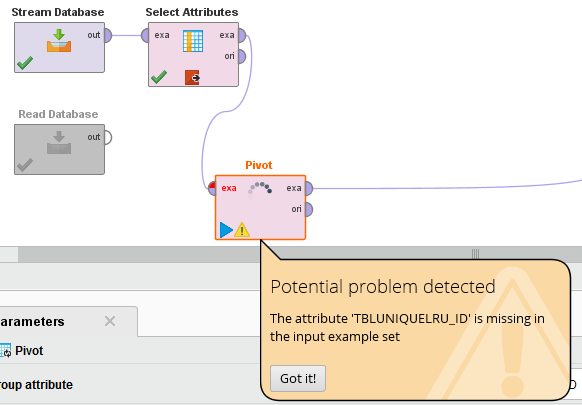# Pivot Operator - "potential problem detected: attribute missing" after Select Attributes

Member Posts: 9Contributor II
edited November 2018 in Help

Hi all,

according to the pivot operator in my data preparation process RapidMiner is experiencing a 'potential problem':I have to stream a big amount of data.

In Select Attributes I chose three attributes. One of them named TBLUNIQUELRU_ID is being missed by the pivot operator although it is contained in the Select Attributes output data:

Data: SimpleExampleSet: 10000000 examples, 3 regular attributes, no special attributes

A breakpoint is set after the second operator and I can confirm, that the attribute is contained in the pivot input example set.

Code:

`<process expanded="true">          <operator activated="true" class="jdbc_connectors:stream_database" compatibility="7.2.001" expanded="true" height="68" name="Stream Database" width="90" x="45" y="34">            <parameter key="connection" value="DB_NAME"/>            <parameter key="table_name" value="ZZ_RM_TEST"/>            <parameter key="recreate_index" value="true"/>          </operator>          <operator activated="true" breakpoints="after" class="select_attributes" compatibility="7.2.001" expanded="true" height="82" name="Select Attributes" width="90" x="179" y="34">            <parameter key="attribute_filter_type" value="subset"/>            <parameter key="attributes" value="|TBLUNIQUELRU_ID|BIT|EVENT"/>          </operator>          <operator activated="true" class="pivot" compatibility="7.2.001" expanded="true" height="82" name="Pivot" width="90" x="246" y="187">            <parameter key="group_attribute" value="TBLUNIQUELRU_ID"/>            <parameter key="index_attribute" value="BIT"/>            <parameter key="consider_weights" value="false"/>            <parameter key="skip_constant_attributes" value="false"/>          </operator>          <connect from_op="Stream Database" from_port="output" to_op="Select Attributes" to_port="example set input"/>          <connect from_op="Select Attributes" from_port="example set output" to_op="Pivot" to_port="example set input"/>          <connect from_op="Pivot" from_port="example set output" to_port="out 1"/>          <portSpacing port="source_in 1" spacing="0"/>          <portSpacing port="sink_out 1" spacing="0"/>          <portSpacing port="sink_out 2" spacing="0"/>        </process>`

Can someone help?

Ina

Tagged:

• Administrator, Moderator, Employee, RapidMiner Certified Analyst, RapidMiner Certified Expert, University Professor Posts: 3,367RM Data Scientist
Solution Accepted

Hi Ina,

propably this is just an issue with the meta data propagation. Isn't there a button to just let it run anyway?

Otherwise I would recommend to switch the metadata propagation to real data by using Process->Synchronize Data with Real Data.

Best,

Martin

- Head of Data Science Services at RapidMiner -
Dortmund, Germany

• Member Posts: 9Contributor II

Hello,

I encounter a problem with attribute recognition with one of the operators. The example set needs to be streamed, since the amount of data is very big (> 30 mio. examples with corporate license and RM Server).

To test and work on the process locally I used a small subset of 10000 rows with the Read Database operator.

Whenever I use Read Database with a subset of 10000 examples - everything is fine.

Whenever I incorporate Stream Database I encounter a 'Potential problem detected' The Pivot operator doesnt recognize one of the crucial fields (an ID-Field which identifies the examples).The code with Stream DB:

` <operator activated="true" class="process" compatibility="7.2.001" expanded="true" name="Process">    <process expanded="true">      <operator activated="true" breakpoints="after" class="subprocess" compatibility="7.2.001" expanded="true" height="82" name="Pivot data" width="90" x="45" y="85">        <process expanded="true">          <operator activated="true" class="jdbc_connectors:stream_database" compatibility="7.2.001" expanded="true" height="68" name="Stream Database" width="90" x="45" y="34">            <parameter key="connection" value="DB_NAME"/>            <parameter key="table_name" value="ZZ_RM_TEST"/>            <parameter key="recreate_index" value="true"/>          </operator>                   <operator activated="false" class="select_attributes" compatibility="7.2.001" expanded="true" height="82" name="Select Attributes (5)" width="90" x="581" y="187">            <parameter key="attribute_filter_type" value="subset"/>          </operator>          <operator activated="true" class="select_attributes" compatibility="7.2.001" expanded="true" height="82" name="Select Attributes (4)" width="90" x="179" y="85">            <parameter key="attribute_filter_type" value="subset"/>            <parameter key="attributes" value="BITID|TBLUNIQUELRU_ID|EVENT"/>          </operator>                   <operator activated="true" breakpoints="after" class="filter_examples" compatibility="7.2.001" expanded="true" height="103" name="Filter Examples" width="90" x="447" y="34">            <list key="filters_list">              <parameter key="filters_entry_key" value="BITID.is_not_missing."/>            </list>          </operator>          <operator activated="true" breakpoints="after" class="pivot" compatibility="7.2.001" expanded="true" height="82" name="Pivot" width="90" x="581" y="34">            <parameter key="group_attribute" value="TBLUNIQUELRU_ID"/>            <parameter key="index_attribute" value="BITID"/>            <parameter key="consider_weights" value="false"/>            <parameter key="skip_constant_attributes" value="false"/>          </operator>          <operator activated="true" class="replace_missing_values" compatibility="7.2.001" expanded="true" height="103" name="Replace Missing Values (3)" width="90" x="715" y="34">            <parameter key="create_view" value="true"/>            <parameter key="attribute_filter_type" value="single"/>            <parameter key="attribute" value="TBLUNIQUELRU_ID"/>            <parameter key="invert_selection" value="true"/>            <parameter key="default" value="value"/>            <list key="columns"/>            <parameter key="replenishment_value" value="N"/>          </operator>          <connect from_op="Stream Database" from_port="output" to_op="Select Attributes (4)" to_port="example set input"/>          <connect from_op="Select Attributes (4)" from_port="example set output" to_op="Filter Examples" to_port="example set input"/>          <connect from_op="Filter Examples" from_port="example set output" to_op="Pivot" to_port="example set input"/>          <connect from_op="Pivot" from_port="example set output" to_op="Replace Missing Values (3)" to_port="example set input"/>          <connect from_op="Replace Missing Values (3)" from_port="example set output" to_port="out 1"/>          <portSpacing port="source_in 1" spacing="0"/>          <portSpacing port="sink_out 1" spacing="0"/>          <portSpacing port="sink_out 2" spacing="0"/>        </process>      </operator>`

I synchronized the meta data with real data as you suggested recently.. Unfortunately it didnt help.

I am trying to avoid to let it run anyway is because I need to know if this is the reason why the processing takes so long. The process loads up to 16 hours, not coming to an end and getting stuck in the Pivot operator. I really would like to know why this potential problem notification appears and how to solve it. Because now it seems that not only the TBLUNIQUELRU_ID is missing in the input example but the attribute BITID as well.Or could you explain to me what the issue with the meta data propagation is about?